## Wednesday, July 28, 2010

### Euler-Maclaurin summation of hypergeometric series

I recently fixed another corner case (it's always the corner cases that are hard!) in the hypergeometric function evaluation code in mpmath.

The difficulty in question concerns the functions pFp-1(...; ...; z), of which the Gauss hypergeometric function 2F1 is an important special case. These functions can be thought of as generalizations of the geometric seriesand the natural logarithmand share the property that the hypergeometric (Maclaurin) series has radius of convergence 1, with a singularity (either algebraic or logarithmic, as above) at z = 1.

Numerical evaluation is fairly easy for z in most of the complex plane. The series for pFp-1(...; ...; z) converges like the geometric series, so direct summation works well for, say, |z| ≤ r 0.9. Likewise, there is an analytic continuation formula which transforms z to 1/z, allowing evaluation for (say) |z| ≥ 1.1.

The remaining shell around the unit circle 0.9 < |z| < 1.1 is the difficult region. In fact, 2F1 can still (essentially) be computed using exact transformations here, but the higher cases remain difficult. As mentioned in an earlier post, mpmath handles this by calling nsum() which applies convergence acceleration to the slowly converging series. In particular, nsum implements the (generalized) Shanks transformation which is extremely efficient for this particular type of series – in fact, it works outside the radius of convergence (Shanks transformation effectively computes a Padé approximant), so it can be used anywhere in the difficult shell.

Still, the Shanks transformation is most efficient when arg(z) = ±π and unfortunately degenerates as arg(z) → 0, i.e. close to z = 1. nsum also implements Richardson extrapolation, which sometimes works at z = 1, but only for special parameter combinations in the hypergeometric series (although those special combinations happen to be quite important – they include the case where the hypergeometric series reduces to a polygamma function or polylogarithm).

Thus, we have the following evaluation strategy for pFp-1:

Blue: direct summation (with respect to z or 1/z)
Yellow: Shanks transformation
Red: ???A commenter suggested trying some other convergence acceleration techniques (like the Levin transformation). But it seems that they still will fail in some cases.

Thus I've finally implemented the Euler-Maclaurin summation formula for the remaining case. The E-M formula differs from other convergence acceleration techniques in that it is based on analyticity of the integrand and does not require a particular rate of convergence. It is implemented in mpmath as sumem(), but requires a little work to apply efficiently.

To apply the E-M formula to a hypergeometric series Σ T(k), the term T(k) must first of all obviously be extended to an analytic function of k, which is done the straightforward way by considering the rising factorials as quotients of gamma functions a(a+1)...(a+k−1) = Γ(a+k) / Γ(a).

We are left with the difficulty of having to integrate T(k) and also to compute n-th order derivatives of this function for the tail expansion in the E-M formula (neither of which can be done in closed form). Fortunately, numerical integration with quad() works very well, but the derivatives remain a problem.

By default, sumem() computes the derivatives using finite differences. Although this works, it gets extremely expensive at high precision for hypergeometric series due to the rapidly increasing cost of evaluating the gamma function as the precision increases. But T(k) is a product of functions that individually can be differentiated logarithmically in closed form (in terms of polygamma functions). I therefore implemented the equivalent of symbolic high-order differentiation for products and the exponential function using generators.

diffs_prod generates the high-order derivatives of a product, given generators for products of individual factors:
>>> f = lambda x: exp(x)*cos(x)*sin(x)>>> u = diffs(f, 1)>>> v = diffs_prod([diffs(exp,1), diffs(cos,1), diffs(sin,1)])>>> next(u); next(v)1.235863336002411.23586333600241>>> next(u); next(v)0.1046589522455960.104658952245596>>> next(u); next(v)-5.96999877552086-5.96999877552086>>> next(u); next(v)-12.4632923122697-12.4632923122697

diffs_exp generates the high-order derivatives of exp(f(x)), given a generator for the derivatives of f(x):
>>> def diffs_loggamma(x):...     yield loggamma(x)...     i = 0...     while 1:...         yield psi(i,x)...         i += 1...>>> u = diffs_exp(diffs_loggamma(3))>>> v = diffs(gamma, 3)>>> next(u); next(v)2.02.0>>> next(u); next(v)1.845568670196931.84556867019693>>> next(u); next(v)2.492929991902692.49292999190269>>> next(u); next(v)3.449965013523673.44996501352367

Actually only differentiation of the exponential function is necessary, which I didn't realize at first, but the product differentiation is still a nice feature to have.

Exponential differentiation amounts to constructing a polynomial of n+1 variables (the derivatives of f), which has P(n) (partition function) ≈ exp(√n) terms. Despite the rapid growth, it is indeed faster to use this approach to differentiate gamma function products at high precision, since the precision can be kept constant. In fact, this should even work in double precision (fp arithmetic) although I think it's broken right now due to some minor bug.

As a result of all this, here are two examples that now work (the results have full accuracy):

>>> mp.dps = 25>>> hyper(['1/3',1,'3/2',2], ['1/5','11/6','41/8'], 1)2.219433352235586121250027>>> hyper(['1/3',1,'3/2',2], ['1/5','11/6','5/4'], 1)+inf>>> eps1 = extradps(6)(lambda: 1 - mpf('1e-6'))()>>> hyper(['1/3',1,'3/2',2], ['1/5','11/6','5/4'], eps1)2923978034.412973409330956

#### Application: roots of polynomials

A neat application is to compute the roots of quintic polynomials. Such roots can be expressed in closed form using hypergeometric functions, as outlined in the Wikipedia article Bring radical. This is more interesting in theory than practice, since polynomial roots can be calculated very efficiently using iterative methods.

In particular, the roots of x5 - x + t are given (in some order) bywhereJust verifying one case:

>>> mp.dps = 25; mp.pretty = True>>> t = mpf(3)>>> for r in polyroots([1,0,0,0,-1,t]):...     print r... -1.341293531690699250693537(0.9790618691253385511579325 - 0.6252190807348055061205923j)(0.9790618691253385511579325 + 0.6252190807348055061205923j)(-0.3084151032799889258111639 + 1.249926913731033699905407j)(-0.3084151032799889258111639 - 1.249926913731033699905407j)>>> -t*hyper(['1/5','2/5','3/5','4/5'], ['1/2','3/4','5/4'], 3125*t**4/256)(-0.9790618691253385511579325 + 0.6252190807348055061205923j)

Now, we can choose t such that the hypergeometric argument becomes unity:

>>> t = root(mpf(256)/3125,4)>>> for r in polyroots([1,0,0,0,-1,t]):...     print r... -1.103842268886161371052433(0.6687403049764220240032331 + 3.167963942396665205386494e-14j)(0.6687403049764220240032331 - 3.172324181973539074536523e-14j)(-0.1168191705333413384770166 + 1.034453571405662514459057j)(-0.1168191705333413384770166 - 1.034453571405662514459057j)>>> -t*hyper(['1/5','2/5','3/5','4/5'], ['1/2','3/4','5/4'], 1)-0.6687403049764220240032331

The last line would previously fail.

Interesting to note is that the value at the singular point z = 1 corresponds to a double root. This also makes polyroots return inaccurate values (note the imaginary parts), a known deficiency that should be fixed...

#### Better methods

In fact, there may be better methods than the Euler-Maclaurin formula. One such approach is to use the Abel-Plana formula, which I've also recently implemented as sumap(). The Abel-Plana formula gives the exact value for an infinite series (subject to some special growth conditions on the analytically extended summand) as a sum of two integrals.

The Abel-Plana formula is particularly useful when the summand decreases like a power of k; for example when the sum is a pure zeta function:

>>> sumap(lambda k: 1/k**2.5, [1,inf])1.34148725725091717975677>>> zeta(2.5)1.34148725725091717975677>>> sumap(lambda k: 1/(k+1j)**(2.5+2.5j), [1,inf])(-3.385361068546473342286084 - 0.7432082105196321803869551j)>>> zeta(2.5+2.5j, 1+1j)(-3.385361068546473342286084 - 0.7432082105196321803869551j)

It actually works very well for the generalized hypergeometric series at the z = 1 singularity (which, as mentioned earlier, is a generalized polygamma function, polylogarithm, or zeta function of integer argument), and near the singularity as well. It should even be slightly faster than the Euler-Maclaurin formula since no derivatives are required. Yet another possibility is to integrate a Mellin-Barnes type contour for the hypergeometric function directly.

But at present, I don't want to modify the existing hypergeometric code because it works and would only get more complicated. Rather, I want to improve nsum so it can handle all of this more easily without external hacks. The numerical integration code should also be improved first, because there are still certain parameter combinations where the hypergeometric function evaluation fails due to slow convergence in the numerical integration (this is due to an implementation issue and not an inherent limitation of the integration algorithm).

## Tuesday, July 27, 2010

### Post Sage Days 24 report

Now that I've overcome the immediate effects of SDWS (Sage Days Withdrawal Syndrome), I should write a brief report about Sage Days 24 which took place last week at the Research Institute for Symbolic Computation (RISC), located in a small town called Hagenberg just outside Linz in Austria. Many thanks for the organizers for inviting me!

Since I'm starting as a Ph.D. student at RISC in October, it was nice to get an early view of the site and meet some current students and staff. The location is quite beautiful, my only complaint being the extremely high temperature last week (they say the weather is more normal most of the year!).

SD24 was titled "Symbolic Computation in Differential Algebra and Special Functions", which pretty accurately describes the topics covered. Some points of interest:

William Stein talked about his vision for Sage (short and long term), including goals for special functions support.

Frédéric Chyzak gave a talk about the Dynamic Dictionary of Mathematical Functions. I really like the approach of starting from a minimal definition of a special function (a differential equation with initial conditions) and generating series expansions, Chebyshev approximations (etc.) algorithmically.

Nico Temme talked about numerical evaluation of special functions, which was familiar grounds to me, but with much food for thought.

I met Manuel Kauers, my future supervisor, who gave a neat presentation of some recent research.

There were many other interesting talks and discussions as well, and I gave a talk about special function evaluation in mpmath (based on my SD23 talk). And of course, I met various other Sage developers and got some coding done as well.

I had hoped to get generalized hypergeometric functions into Sage during SD24. There is now a patch, but it still needs some more work.

I also implemented parabolic cylinder functions (PCFs) in mpmath, the last remaining chapter of hypergeometric functions listed in the DLMF (mpmath now covers chapters 1-20, 22, 24-25, partially 26-27, and 33). Code commit here.

Technically, parabolic cylinder functions are just scaled (linear combinations of) confluent hypergeometric functions or Whittaker functions, but they are a bit tricky to compute due to cancellation and branch cuts.

An example from Nico Temme's talk was to evaluate Dν(z) with ν = -300.14 and z = 300.15 (Maple 13 takes 5 seconds to give an answer that has the wrong sign and is off by 692 orders of magnitude). The new pcfd function in mpmath instantaneously (in about a millisecond) gives the correct result:
>>> pcfd(-300.14, 300.15)6.83322814925713e-10526>>> mp.dps = 30>>> pcfd('-300.14', '300.15')6.83322814923312844480669009487e-10526

All the curve plots from DLMF 12.3 can be reproduced as follows:
f1 = lambda x: pcfu(0.5,x)f2 = lambda x: pcfu(2,x)f3 = lambda x: pcfu(3.5,x)f4 = lambda x: pcfu(5,x)f5 = lambda x: pcfu(8,x)plot([f1,f2,f3,f4,f5], [-3,3], [0,3])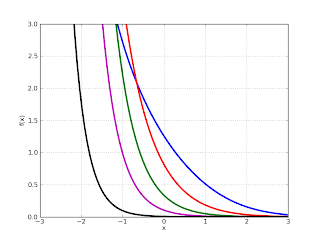f1 = lambda x: pcfv(0.5,x)f2 = lambda x: pcfv(2,x)f3 = lambda x: pcfv(3.5,x)f4 = lambda x: pcfv(5,x)f5 = lambda x: pcfv(8,x)plot([f1,f2,f3,f4,f5], [-3,3], [-3,3])f1 = lambda x: pcfu(-0.5,x)f2 = lambda x: pcfu(-2,x)f3 = lambda x: pcfu(-3.5,x)f4 = lambda x: pcfu(-5,x)plot([f1,f2,f3,f4], [-8,8], [-6,6])f1 = lambda x: pcfv(-0.5,x)f2 = lambda x: pcfv(-2,x)f3 = lambda x: pcfv(-3.5,x)f4 = lambda x: pcfv(-5,x)plot([f1,f2,f3,f4], [-8,8], [-6,6])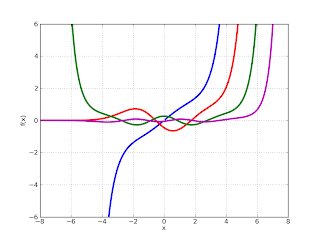f1 = lambda x: pcfu(-8,x)f2 = lambda x: pcfv(-8,x)*gamma(0.5-(-8))f3 = lambda x: hypot(f1(x), f2(x))plot([f1,f2,f3], [-6,6], [-120,120])f1 = lambda x: diff(pcfu,(-8,x),(0,1))f2 = lambda x: diff(pcfv,(-8,x),(0,1))*gamma(0.5-(-8))f3 = lambda x: hypot(f1(x), f2(x))plot([f1,f2,f3], [-6,6], [-220,220])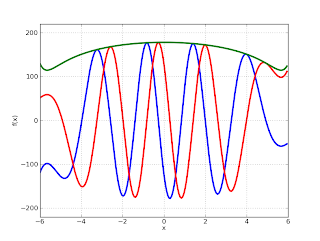A little more information can be found in the mpmath documentation section for PCFs.

Thanks again to the NSF grant enabling this work.

## Saturday, July 10, 2010

### Sage Days 23, and Bessel function zeros

Yesterday I came back home from Sage Days 23 in Leiden. I don't really have time to write a detailed travel report right now, but overall it was very, very nice. Those interested can check out William Stein's photos (1, 2, 3, 4) of the event (I can be seen in a few of the photos, wearing a blue t-shirt during days 1-3 and a yellow t-shirt during day 4).

I didn't get quite as much coding done as I probably had time for (in part because I had a talk to prepare, and in part because I spent a bunch of time playing with MPIR and FLINT 2 and gaining some insights but otherwise not accomplishing much productive).

The code I did write includes a complete Cython implementation of real exp (giving up to a 7x speedup) and complex sqrt for mpmath, as well as code for fast evaluation of the gamma function at rational arguments. I will submit the Cython code to Sage soon; possibly after rewriting the remaining power code in Cython as well.

Anyway, prompted by a recent question on sage-support (and because I finally got inspiration to do it while at the airport), I've now implemented Bessel function zeros in mpmath.

Bessel function zeros are very important in applied mathematics because they are needed for Fourier–Bessel series, used to solve partial differential equations in cylindrical coordinates. I'd like to write down a concrete computational example, but it'd be slightly involved and I don't really have time right now. Perhaps in a later blog post.

The code permits computing the m-th nonnegative simple zero of Jν(z), J'ν(z) Yν(z), or Y'ν(z) for any positive integer index m and real order ν ≥ 0. One can use it for large m, large ν, or both:
>>> besseljzero(1,100)314.9434728377671624580656>>> besseljzero(100,1)108.836165898409774363098>>> besseljzero(100,100)459.5295465754674695983379

A word of caution: I've chosen the convention Abramowitz & Stegun of including z = 0 for J'0, which is incompatible with SciPy and the Mathematica BesselZeros package. The A&S convention makes much more sense to me because it makes the zeros a continuous function of the order. This case (ν=0, derivative=1) should be the only incompatibility.

It's a bit tricky to compute the zeros of Bessel functions, because (as far as I know) no globally valid approximations are known; not any reasonably simple ones anyway.

Asymptotic expansions are known with respect to either the order or the index. In my implementation, I've chosen to simply use the asymptotic expansion with respect to the index n. If the n is too small, the code simply chooses a larger index m for which the asymptotic expansion does work, and then isolates all the zeros between 0 and the m-th by counting sign changes. By caching this result, all the consecutive zeros for the same function order can then be computed quickly as well.

This approach is simple and quite robust (up to any silly implementation errors on my part), although the code could certainly be optimized more for large orders.

The following graphic shows the derivative of Y50(z) with its zeros marked:A neat property of Bessel functions is that they can be expanded as infinite products over their zeros: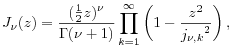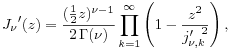These products converge slowly, but work with the aid of convergence acceleration:
>>> v, z = mpf(3), mpf(0.75)>>> (z/2)**v/gamma(v+1)*...     nprod(lambda k: 1-(z/besseljzero(v,k))**2, [1,inf])...0.008484383423274108843927552>>> besselj(v,z)0.008484383423274108843927552>>> (z/2)**(v-1)/2/gamma(v)*...     nprod(lambda k: 1-(z/besseljzero(v,k,1))**2, [1,inf])...0.0331364636065541211306414>>> besselj(v,z,1)0.0331364636065541211306414

I will do more work on Bessel functions in mpmath and Sage, so watch for future updates.

## Friday, July 2, 2010

### Symbolic infinite series

I've written a little module for symbolically evaluating hypergeometric infinite series. Technically, a series is hypergeometric if the ratio between successive terms is a rational function of the index k; more intuitively, most "nice-looking" series (such as power series of most special functions) involving powers, factorials, gamma functions, binomial coefficients (etc.) are hypergeometric.

The code currently uses SymPy, but should be possible to convert to Sage's symbolics (it mostly requires basic pattern matching, and evaluation of standard special functions). Perhaps "rewrite" is a better word than "convert" because the current code is a messy hack. It's buggy, and only handles special cases in places where a general approach could be used (such as for rational functions).

The basic idea behind the code is to perform the evaluation in two steps. Firstly, the given series is converted into canonical form. I've used the standard generalized hypergeometric series pFq. This is not always an optimal representation, but usually does the job.

In the second step, parameter transformations and table lookups are used to reduce the pFq series into elementary (or simpler) functions when possible. If this step fails, the pFq function is returned, giving a closed-form solution that can be manipulated or evaluated numerically.

The second step is the most difficult, because the transformation algorithms that need to be used for hypergeometric functions are very complicated in general (and there are all kinds of special cases). So far I've only implemented some simple transformations. For the final lookup stage, it would be straightforward to also implement expansion into Bessel functions, incomplete gamma functions, etc.

Below is a sample of some working test cases, with numerical evaluation of both the evaluated result and the original series as verification. Some of the sums don't quite simplify fully, i.e. there is an unevaluated pFq function left. The formulas were mostly generated with SymPy's latex function, so they are not always as pretty as could be.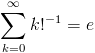>>> hypsum(1 / fac(k), k)E>>> _.evalf()2.71828182845905>>> mpmath.nsum(lambda k: 1 / mpmath.fac(k), [0,mpmath.inf])2.71828182845905>>> hypsum(k**6 / fac(k), k)203*E>>> _.evalf()551.811211177186>>> mpmath.nsum(lambda k: k**6 / mpmath.fac(k), [0,mpmath.inf])551.811211177186>>> hypsum(4 * d * k * z**(k+2) / fac(k), k)4*d*z**3*exp(z)>>> _.evalf(subs={d:'1/2',z:'1/4'})0.0401257942714919>>> mpmath.nsum(lambda k: 4 * 0.5 * k * 0.25**(k+2) / mpmath.fac(k), [0,mpmath.inf])0.0401257942714919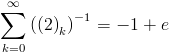>>> hypsum(1 / rf(2,k), k)-1 + E>>> _.evalf()1.71828182845905>>> mpmath.nsum(lambda k: 1 / mpmath.rf(2,k), [0,mpmath.inf])1.71828182845905>>> simplify(hypsum(k**3 * z**(k) / fac(k), k))z*exp(z) + z**3*exp(z) + 3*z**2*exp(z)>>> _.evalf(subs={d:'1/2',z:'1/4'})0.581824016936633>>> mpmath.nsum(lambda k: k**3 * 0.25**(k) / mpmath.fac(k), [0,mpmath.inf])0.581824016936633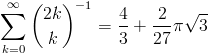>>> simplify(hypsum(1 / binomial(2*k,k), k))4/3 + 2*pi*3**(1/2)/27  >>> _.evalf()1.73639985871872>>> mpmath.nsum(lambda k: 1 / mpmath.binomial(2*k,k), [0,mpmath.inf])1.73639985871872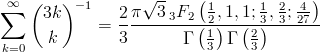>>> hypsum(1 / binomial(3*k,k), k)2*pi*3**(1/2)*3F2([1/2, 1, 1], [1/3, 2/3], 4/27)/(3*gamma(1/3)*gamma(2/3))>>> _.evalf()1.41432204432182>>> mpmath.nsum(lambda k: 1 / mpmath.binomial(3*k,k), [0,mpmath.inf])1.41432204432182>>> hypsum(k**4 / binomial(2*k,k), k)32/3 + 238*pi*3**(1/2)/243>>> _.evalf()15.9961018356512>>> mpmath.nsum(lambda k: k**4 / mpmath.binomial(2*k,k), [0,mpmath.inf])15.9961018356512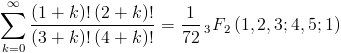>>> hypsum(fac(k+1)*fac(k+2)/fac(k+3)/fac(k+4), k)3F2([1, 2, 3], [4, 5], 1)/72>>> _.evalf()0.0217325998184402>>> mpmath.nsum(lambda k: mpmath.fac(k+1)*mpmath.fac(k+2)/mpmath.fac(k+3)/mpmath.fac(k+4), [0,mpmath.inf])0.0217325998184402>>> hypsum(1/((3+k)**2*(8+6*k+k**2)), k)3F2([1, 2, 3], [4, 5], 1)/72>>> _.evalf()0.0217325998184402>>> mpmath.nsum(lambda k: 1/((3+k)**2*(8+6*k+k**2)), [0,mpmath.inf])0.0217325998184402>>> hypsum(fac(2*k+1)/(fac(2*k+2)*(k+1)), k)pi**2/12>>> _.evalf()0.822467033424113>>> mpmath.nsum(lambda k: mpmath.fac(2*k+1)/(mpmath.fac(2*k+2)*(k+1)), [0,mpmath.inf])0.822467033424113>>> hypsum(fac(2*k+1)/(fac(3*k+2)), k)2*pi*3**(1/2)*2F2([1, 3/2], [4/3, 5/3], 4/27)/(27*gamma(4/3)*gamma(5/3))>>> _.evalf()0.553106730441975>>> mpmath.nsum(lambda k: mpmath.fac(2*k+1)/(mpmath.fac(3*k+2)), [0,mpmath.inf])0.553106730441975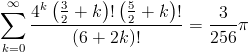>>> hypsum(4**k*fac(k+R32)*fac(k+R52)/(fac(2*k+6)), k)3*pi/256>>> _.evalf()0.0368155389092554>>> mpmath.nsum(lambda k: 4**k*mpmath.fac(k+1.5)*mpmath.fac(k+2.5)/(mpmath.fac(2*k+6)), [0,mpmath.inf], method='e')0.0368155389092358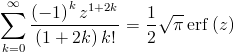>>> hypsum((-1)**k * z**(2*k+1) / fac(k) / (2*k+1), k)pi**(1/2)*erf(z)/2>>> _.evalf(subs={d:'1/2',z:'1/4'})0.244887887180256>>> mpmath.nsum(lambda k: (-1)**k * 0.25**(2*k+1) / mpmath.fac(k) / (2*k+1), [0,mpmath.inf])0.244887887180256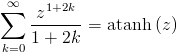>>> hypsum(z**(2*k+1) / (2*k+1), k)atanh(z)>>> _.evalf(subs={d:'1/2',z:'1/4'})0.255412811882995>>> mpmath.nsum(lambda k: 0.25**(2*k+1) / (2*k+1), [0,mpmath.inf])0.255412811882995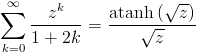>>> hypsum(z**k / (2*k+1), k)atanh(z**(1/2))/z**(1/2)>>> _.evalf(subs={d:'1/2',z:'1/4'})1.09861228866811>>> mpmath.nsum(lambda k: 0.25**k / (2*k+1), [0,mpmath.inf])1.09861228866811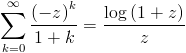>>> hypsum((-z)**k / (k+1), k)log(1 + z)/z>>> _.evalf(subs={d:'1/2',z:'1/4'})0.892574205256839>>> mpmath.nsum(lambda k: (-0.25)**k / (k+1), [0,mpmath.inf])0.892574205256839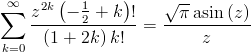>>> hypsum(fac(k-R12)/((1+2*k)*fac(k))*z**(2*k), k)pi**(1/2)*asin(z)/z>>> _.evalf(subs={d:'1/2',z:'1/4'})1.79145636509746>>> mpmath.nsum(lambda k: mpmath.fac(k-0.5)/((1+2*k)*mpmath.fac(k))*0.25**(2*k), [0,mpmath.inf])1.79145636509746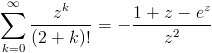>>> hypsum(z**k / fac(k+2), k)-(1 + z - exp(z))/z**2>>> _.evalf(subs={d:'1/2',z:'1/4'})0.544406667003864>>> mpmath.nsum(lambda k: 0.25**k / mpmath.fac(k+2), [0,mpmath.inf])0.544406667003864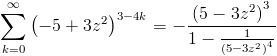>>> hypsum((3*z**2-5)**(-4*k+3), k)-(5 - 3*z**2)**3/(1 - 1/(5 - 3*z**2)**4)>>> _.evalf(subs={d:'1/2',z:'1/4'})-111.666432272586>>> mpmath.nsum(lambda k: (3*0.25**2-5)**(-4*k+3), [0,mpmath.inf])-111.666432272586>>> hypsum(z**(2*k+1) / fac(2*k+1), k)sinh(z)>>> _.evalf(subs={d:'1/2',z:'1/4'})0.252612316808168>>> mpmath.nsum(lambda k: 0.25**(2*k+1) / mpmath.fac(2*k+1), [0,mpmath.inf])0.252612316808168>>> hypsum((-1)**k * z**(2*k+1) / fac(2*k+1), k)sin(z)>>> _.evalf(subs={d:'1/2',z:'1/4'})0.247403959254523>>> mpmath.nsum(lambda k: (-1)**k * 0.25**(2*k+1) / mpmath.fac(2*k+1), [0,mpmath.inf])0.247403959254523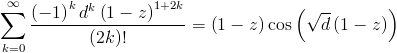>>> hypsum((-1)**k * d**k * (1-z)**(2*k+1) / fac(2*k), k)(1 - z)*cos(d**(1/2)*(1 - z))>>> _.evalf(subs={d:'1/2',z:'1/4'})0.646980115568008>>> mpmath.nsum(lambda k: (-1)**k * 0.5**k * (1-0.25)**(2*k+1) / mpmath.fac(2*k), [0,mpmath.inf])0.646980115568008>>> hypsum(k * z**(2*k) / fac(2*k+1), k)cosh(z)/2 - sinh(z)/(2*z)>>> _.evalf(subs={d:'1/2',z:'1/4'})0.0104819163234500>>> mpmath.nsum(lambda k: k * 0.25**(2*k) / mpmath.fac(2*k+1), [0,mpmath.inf])0.01048191632345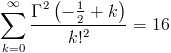>>> hypsum(gamma(k-R12)**2/(fac(k)**2), k)16>>> _.evalf()16.0000000000000>>> mpmath.nsum(lambda k: mpmath.gamma(k-0.5)**2/(mpmath.fac(k)**2), [0,mpmath.inf])16.0>>> hypsum(1/(k+1)**2, k)pi**2/6>>> _.evalf()1.64493406684823>>> mpmath.nsum(lambda k: 1/(k+1)**2, [0,mpmath.inf])1.64493406684823>>> hypsum(k/(k+1)**3, k)-zeta(3) + pi**2/6>>> _.evalf()0.442877163688632>>> mpmath.nsum(lambda k: k/(k+1)**3, [0,mpmath.inf])0.442877163688632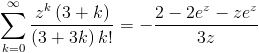>>> simplify(hypsum((3+k)/(3+3*k)*z**k/fac(k), k))-(2 - 2*exp(z) - z*exp(z))/(3*z)>>> _.evalf(subs={d:'1/2',z:'1/4'})1.18540958339656>>> mpmath.nsum(lambda k: (3+k)/(3+3*k)*0.25**k/mpmath.fac(k), [0,mpmath.inf])1.18540958339656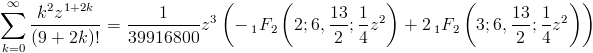>>> hypsum(k**2 * z**(2*k+1) / fac(2*k+9), k)z**3*(-1F2(, [6, 13/2], z**2/4) + 2*1F2(, [6, 13/2], z**2/4))/39916800>>> _.evalf(subs={d:'1/2',z:'1/4'})3.92066920165299e-10>>> mpmath.nsum(lambda k: k**2 * 0.25**(2*k+1) / mpmath.fac(2*k+9), [0,mpmath.inf])3.92066920165299e-10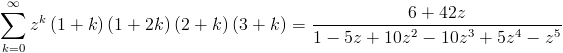>>> simplify(hypsum((k+1)*(k+2)*(k+3)*(1+2*k)*z**k, k))(6 + 42*z)/(1 - 5*z + 10*z**2 - 10*z**3 + 5*z**4 - z**5)>>> _.evalf(subs={d:'1/2',z:'1/4'})69.5308641975309>>> mpmath.nsum(lambda k: (k+1)*(k+2)*(k+3)*(1+2*k)*0.25**k, [0,mpmath.inf])69.5308641975309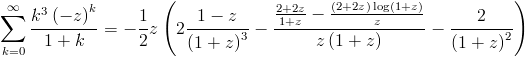>>> hypsum(k**3 * (-z)**k / (k+1), k)-z*(2*(1 - z)/(1 + z)**3 - ((2 + 2*z)/(1 + z) - (2 + 2*z)*log(1 + z)/z)/(z*(1 + z)) - 2/(1 + z)**2)/2>>> _.evalf(subs={d:'1/2',z:'1/4'})-0.0285742052568390>>> mpmath.nsum(lambda k: k**3 * (-0.25)**k / (k+1), [0,mpmath.inf])-0.028574205256839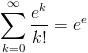>>> hypsum(E**k / fac(k), k)exp(E)>>> _.evalf()15.1542622414793>>> mpmath.nsum(lambda k: mpmath.e**k / mpmath.fac(k), [0,mpmath.inf])15.1542622414793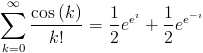>>> hypsum(cos(k) / fac(k), k)exp(exp(I))/2 + exp(exp(-I))/2>>> _.evalf()1.14383564379164 + .0e-20*I>>> mpmath.nsum(lambda k: mpmath.cos(k) / mpmath.fac(k), [0,mpmath.inf])1.14383564379164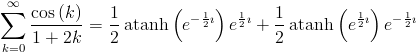>>> hypsum(cos(k) / (2*k+1), k)atanh(exp(-I/2))*exp(I/2)/2 + atanh(exp(I/2))*exp(-I/2)/2>>> _.evalf()0.975556628913311>>> mpmath.nsum(lambda k: mpmath.cos(k) / (2*k+1), [0,mpmath.inf])0.975556628913311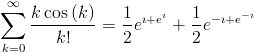>>> simplify(hypsum(cos(k) * k / fac(k), k))exp(I + exp(I))/2 + exp(-I + exp(-I))/2>>> _.evalf()-0.458967373729452 + .0e-20*I>>> mpmath.nsum(lambda k: mpmath.cos(k) * k / mpmath.fac(k), [0,mpmath.inf])-0.458967373729452>>> hypsum((-1)**k / (2*k+1), k)pi/4>>> _.evalf()0.785398163397448>>> mpmath.nsum(lambda k: (-1)**k / (2*k+1), [0,mpmath.inf])0.785398163397448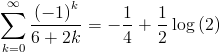>>> hypsum((-1)**k / (2*k+6), k)-1/4 + log(2)/2>>> _.evalf()0.0965735902799727>>> mpmath.nsum(lambda k: (-1)**k / (2*k+6), [0,mpmath.inf])0.0965735902799727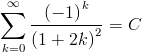>>> hypsum((-1)**k / (2*k+1)**2, k)Catalan>>> _.evalf()0.915965594177219>>> mpmath.nsum(lambda k: (-1)**k / (2*k+1)**2, [0,mpmath.inf])0.915965594177219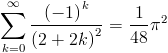>>> hypsum((-1)**k / (2*k+2)**2, k)pi**2/48>>> _.evalf()0.205616758356028>>> mpmath.nsum(lambda k: (-1)**k / (2*k+2)**2, [0,mpmath.inf])0.205616758356028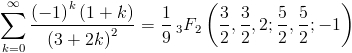>>> hypsum((-1)**k * (k+1) / (2*k+3)**2, k)3F2([3/2, 3/2, 2], [5/2, 5/2], -1)/9>>> _.evalf()0.0652837153898853>>> mpmath.nsum(lambda k: (-1)**k * (k+1) / (2*k+3)**2, [0,mpmath.inf])0.0652837153898854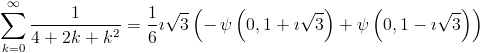>>> hypsum(1/(k**2+2*k+4), k)I*3**(1/2)*(-polygamma(0, 1 + I*3**(1/2)) + polygamma(0, 1 - I*3**(1/2)))/6>>> _.evalf()0.740267076581851>>> mpmath.nsum(lambda k: 1/(k**2+2*k+4), [0,mpmath.inf])0.740267076581851

This work was possible thanks to the support of NSF grant DMS-0757627, which is gratefully acknowledged.